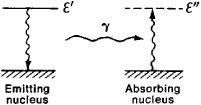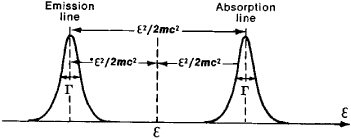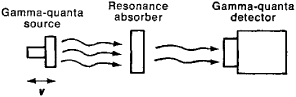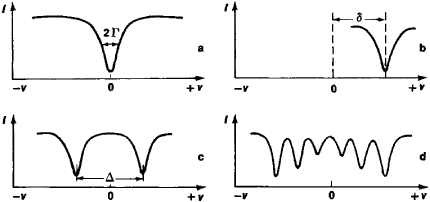# Mössbauer Effect

(redirected from Mossbauer effect)
Also found in: Dictionary.
Related to Mossbauer effect: Mossbauer spectroscopy

## Mössbauer effect

Recoil-free gamma-ray resonance absorption. The Mössbauer effect, also called nuclear gamma resonance fluorescence, has become the basis for a type of spectroscopy which has found wide application in nuclear physics, structural and inorganic chemistry, biological sciences, the study of the solid state, and many related areas of science.

The fundamental physics of this effect involves the transition (decay) of a nucleus from an excited state of energy Ee to a ground state of energy Eg with the emission of a gamma ray of energy Eγ. If the emitting nucleus is free to recoil, so as to conserve momentum, the emitted gamma ray energy is Eγ = (Ee - Eg) - Er, where Er is the recoil energy of the nucleus. Tlie magnitude of Er is given classically by the relationship, where m is the mass of the recoiling atom and c is the speed of light. Since Er is a positive number, the Eγ will always be less than the difference Ee - Eg, and if the gamma ray is now absorbed by another nucleus, its energy is insufficient to promote the transition from Eg to Ee.

In 1957 R. L. Mössbauer discovered tnat if the emitting nucleus is held by strong bonding forces in the lattice of a solid, the whole lattice takes up the recoil energy, and the mass in the recoil energy equation given above becomes the mass of the whole lattice. Since this mass typically corresponds to that of 1010 to 1020 atoms, the recoil energy is reduced by a factor of 10-10 to 10-20, with the important result that Er 0 so that Eγ = Ee - Eg; that is, the emitted gamma-ray energy is exactly equal to the difference between the nuclear ground-state energy and the excited-state energy. Consequently, absorption of this gamma ray by a nucleus which is also firmly bound to a solid lattice can result in the “pumping” of the absorber nucleus from the ground state to the excited state. See Energy level (quantum mechanics), Excited state, Gamma rays

In a typical Mössbauer experiment the radioactive source is mounted on a velocity transducer which imparts a smoothly varying motion (relative to the absorber, which is held stationary), up to a maximum of several centimeters per second, to the source of the gamma rays. These gamma rays are incident on the material to be examined (the absorber). Some of the gamma rays are absorbed and reemitted in all directions, while the remainder of the gamma rays traverse the absorber and are registered in an appropriate detector.

A typical display of a Mössbauer spectrum, which is the result of many repetitive scans through the velocity range of the transducer, is shown in the illustration. In certain nuclides the Mössbauer resonance line displays splitting that arises from the coupling of the nuclear electric quadrupole moment with the electric field gradient or of the nuclear magnetic dipole moment with the magnetic field at the nucleus, providing information on the magnitude of these interactions.

Mössbauer effect experiments have been used to elucidate problems in a very wide range of scientific disciplines. Applications include the measurement of nuclear magnetic and quadrupole moments and of excited-state lifetimes involved in the nuclear decay process; study of the chemical consequences of nuclear decay; study of the nature of magnetic interactions in iron-containing alloys and of the dependence of the magnetic field in these alloys on various parameters; study of the effects of high pressure on chemical properties of materials; investigation of the relationship between chemical composition and structure on the one hand and the superconductive transition on the other; investigation of the structure of compounds; and study of the structure and bonding properties of metal atoms in complex biological molecules.

## Mössbauer Effect

the resonance absorption of gamma radiation by atomic nuclei, which is observed when the source and the absorber of the radiation are solids and the energy of the gamma quanta is low (⋍ 150 kiloelectron volts [keV]). The Mössbauer effect is sometimes called recoil-free resonance absorption or nuclear gamma resonance.Figure 1. Schematic representation of emission and resonance absorption of gamma quanta. The emitting and absorbing nuclei are identical; therefore, the energieε’ and ε” of their excited states are equal.

When a substance is irradiated with gamma radiation, in addition to the usual interaction processes it is also possible to have resonance absorption of gamma quanta by the nuclei. In the latter process, a gamma quantum disappears and the nucleus is excited, that is, the nucleus is raised to a state with greater internal energy. This phenomenon is analogous to the resonance absorption of light quanta (photons) by atoms. A necessary condition for resonance absorption is that the energy which the quantum gives up in the excitation of the nucleus must be exactly equal to the energy of the quantum transition, that is, the difference between the internal energies of the nucleus in the excited and ground states. At first glance, this condition would seem to be automatically satisfied when the emitting and absorbing nuclei are of the same kind (Figure 1). However, a gamma quantum with energy S has momentum p = £/c (where c is the velocity of light), and, according to the law of conservation of momentum, when a nucleus emits or absorbs a quantum, the nucleus experiences a recoil. A nucleus of mass m that emits a quantum acquires the momentum p,2 thus obtaining the kinetic energy ΔS = p2/2m = £2/2mc2. Thus, part of the energy of the gamma transition is transformed into kinetic energy of the nucleus, and the energy of the emitted quantum is less than the total energy of the gamma transition by the amount ΔS. The same energy ΔS is also transmitted to a free (stationary) nucleus during absorption. Therefore, in order to attain resonance, a gamma quantum incident on a nucleus must have an energy that is greater than the transition energy by the amount ΔS. As a result, the emission and absorption lines are shifted with respect to each other by the amount 2 ΔS = &2/mc2 (Figure 2).Figure 2. Shift of emission and absorption lines with respect to energy £ of gamma transition; Γ is the line width

The quantity ΔS is an extremely small fraction of the transition energy S, but ΔS always greatly exceeds the line width of the radiation. Therefore, the emission and absorption lines overlap only slightly and the probability of resonance absorption of gamma quanta is extremely small. For example, for 14.4-keV gamma radiation (of the 57Fe nucleus), the quantity ΔS ⋍ 2 X 10−3 eV, whereas the natural line width is Γ = 4.6 X 10−9 eV.

Real nuclei are not free; however, in most cases, the energy loss ΔS owing to recoil is nearly the same as that for the case of free stationary nuclei that was considered above. Because of Doppler broadening, the widths of the gamma-radiation lines usually greatly exceed the natural line widths Γ; the Doppler broadening is due to the thermal motion of the atoms. At room temperature, however, the overlapping of the emission and absorption lines still remains very small; in observing resonance absorption of light by atoms, the recoil energy is small owing to the low energy of the photon, and the shifts of the emission and absorption lines are insignificant.

In order to make the resonance absorption of gamma quanta observable, the overlapping of the emission and absorption lines must be artificially increased. The shift in the lines owing to the Doppler effect when the emitting and the absorbing nuclei are moving toward each other is used for this purpose. In experiments that have been carried out, the necessary velocity (hundreds of meters per second) was imparted by one of three methods: (1) by mechanical displacement of the source or the absorber, (2) by the recoil experienced by the nucleus if the radiation of the gamma quantum is preceded by alpha or beta decay, or, (3) by heating the source and the absorber up to a high temperature.

In 1958, R. Mössbauer observed that for nuclei in solids, emission and absorption of gamma quanta without an energy loss owing to recoil may occur at low gamma-transition energies. Unshifted lines with an energy exactly equal to the energy of the gamma transition are observed in the emission and absorption spectra; the widths of these lines are equal (or are extremely close) to the natural width Γ. In this case, the emission and absorption lines overlap, making it possible to observe resonance absorption of gamma quanta.

This phenomenon, which is called the Mössbauer effect, is due to the collective character of the motion of the atoms in a solid. As a result of the strong interaction of the atoms in solids, the recoil energy is not imparted to an individual nucleus but is converted into vibrational energy of the crystal lattice; in other words, the recoil leads to the production of phonons. However, if the recoil energy (per nucleus) is less than the average phonon energy that is characteristic of the given crystal, then the recoil will not lead to the production of a phonon for every event. In such “phononfree” cases, the recoil does not change the internal energy of the crystal. However, the kinetic energy acquired by the crystal as a whole as it absorbs the recoil momentum of the gamma quantum is negligibly small. In this case, the transfer of momentum will not be accompanied by a transfer of energy; therefore, the position of the emission and absorption lines will correspond precisely to the transition energy.

The probability of this process runs as high as several tens of percent if the energy of the gamma transition is sufficiently small; in practice, the Mössbauer effect is observed only when £ ⋍ 150 keV (the probability of phonon production upon recoil increases with increasing £). The probability of the Mössbauer effect is also strongly temperature-dependent. In order to observe the Mössbauer effect, the source of the gamma radiation and the absorber often must be cooled to the temperature of liquid nitrogen or liquid helium; however, for very-low-energy gamma transitions (for example, £ = 14.4 keV for the gamma transition of the 57Fe nucleus and & = 23.8 keV for the gamma transition of the 119Sn nucleus), the Mössbauer effect can be observed up to temperatures exceeding 1000°C. All other conditions being equal, the stronger the interaction of atoms in the solid—that is, the greater the energy of the phonons—the higher will be the probability of the Mössbauer effect. Therefore, the greater the Debye temperature of the crystal, the higher will be the probability of the Mössbauer effect.

An extremely small line width is an intrinsic property of recoil-free resonance absorption; it is this property that has transformed the Mössbauer effect from a laboratory experiment into an important method of research. The ratio of the line width to the energy of the gamma quantum in the Mössbauer effect is, for example, ⋍3 X 10−13 for 57Fe nuclei and ⋍5.2 X 10−16 for 67Zn nuclei. Such small line widths have not been achieved even in the gas laser, which is a source of the narrowest lines in the infrared and visible regions of the electromagnetic spectrum. Using the Mössbauer effect, it has proved to be possible to observe processes in which the energy of a gamma quantum differs by an extremely small amount (⋍Γ or even small fractions of Γ) from the transition energy of the nuclei of the absorber. Such energy changes lead to a relative shift of the emission and absorption lines. This brings about a change in the value of resonance absorption that can be measured.

The possibilities of methods based on the use of the Mössbauer effect is well illustrated by an experiment in which the change in the frequency of a quantum of electromagnetic radiation in the earth’s gravitational field, predicted by relativity theory, was measured under laboratory conditions. In this experiment, carried out in 1959 by R. Pound and G. Rebka, Jr., in the United States, the gamma-radiation source was placed at a height of 22.5 m above the absorber. The corresponding change in the gravitational potential should lead to a relative change in the energy of the gamma quantum equal to 2.5 X 10−15. The shift in the emission and absorption lines proved to agree with the theory.

Shifts and splittings of the energy levels of the nucleus, and consequently changes in the transition energy, can occur under the influence of internal electric and magnetic fields acting on the nuclei of atoms in solids, and also under the influence of external factors, such as pressure and external magnetic fields. Since the values of these changes are related to the microscopic structure of solids, the study of the shift of emission and absorption lines makes it possible to obtain information on the structure of solids. These shifts can be measured with Mössbauer spectrometers (Figure 3). If the gamma quanta are emitted by a source moving with velocity v with respect to the absorber, then as a result of the Doppler effect the energy of the gamma quanta incident on the absorber changes by the amount £v/c. (For the nuclei usually used in observing the Mössbauer effect, a change in the energy £ by the amount Γ corresponds to values of the velocity v ranging from 0.2 to 10 mm/sec.) The value of the velocity for which the emission and absorption lines are in exact resonance, that is, when the absorption is a maximum, is found by measuring the dependence of the magnitude of resonance absorption on the value of v (the Mössbauer resonance-absorption spectrum). The displacement Δ£ between the emission and absorption lines for a stationary source and a stationary absorber is determined from the value of v.Figure 3. Simplified schematic diagram of Mössbauer spectrometer; gamma-quanta source is set in reciprocating motion with velocity v with respect to absorber by a mechanical or electrodynamic device. Dependence of flux intensity of gamma quanta passing through absorber on velocity v is measured with a gamma-radiation detector.

An absorption spectrum that consists of a single line is illustrated in Figure 4, a: the emission and absorption lines are not shifted with respect to each other, that is, exact resonance exists for v = 0. The shape of the observed line can be described sufficiently accurately by a Lorentz curve (or the Breit-Wigner formula) with a width at half-height of 2Γ. Such a spectrum is observed only when the source and the absorber are made of chemically identical materials and when the nuclei of the atoms in these materials are acted upon by neither a magnetic nor an inhomogeneous electric field. In most cases, several lines (a hyperfine structure) that are due to the interaction of the atomic nuclei with extranuclear electric and magnetic fields are observed in the spectrum. The characteristics of the hyperfine structure depend both on the properties of the nuclei in the ground state and in the excited state and on distinguishing features of the structure of the solids containing the radiating and absorbing nuclei.

Electric-monopole, electric-quadrupole, and magnetic-dipole interactions are the most important types of interactions of an atomic nucleus with an extranuclear field. An electric-monopole interaction is the interaction of a nucleus with the electrostatic field generated in the vicinity of the nucleus by the surrounding electrons; this type of interaction leads to a line shift 8 in the absorption spectrum (Figure 4, b) if the source and the absorber are chemically different or if the distribution of the electric charge in the nucleus is different in the ground state and in the excited state. This isomeric or chemical shift is proportional to the electron density in the vicinity of the nucleus, and the magnitude of this shift is an important characteristic of the chemical bonding of the atoms in solids. Such features as the ionic and covalent character of the chemical bond, the effective charges of atoms in chemical compounds, and the electronegativity of the atoms making up molecules can be assessed from the magnitude of this shift. Investigation of chemical shifts also makes it possible to obtain data on the charge distribution in atomic nuclei.Figure 4. Mössbauer resonance absorption spectra of gamma quanta: / is the flux intensity of gamma quanta passing through absorber and v the velocity of gamma-quanta source, (a) Individual emission and absorption lines that are not shifted with respect to each other when v — 0. (b) Isomeric or chemical line shift; shift Δ is proportional to electron density in vicinity of nucleus and depends on features of chemical bond of atoms in solid, (c) Quadrupole doublet that is observed for such isotopes as 57Fe, 119Sn, and 125Te; magnitude of splitting Δ is proportional to electric-field gradient in vicinity of nucleus, (d) Magnetic hyperfine structure observed in absorption spectra for magnetically ordered materials; distance between components of structure is proportional to intensity of magnetic field acting on nuclei of atoms in solid.

The electric-quadrupole interaction—the interaction of the nuclear quadrupole moment with an inhomogeneous electric field—leads to a splitting of the nuclear levels, as a result of which we observe not one line but several lines in the absorption spectrum. For example, two lines (a quadrupole doublet, Figure 4,c) are observed in the absorption spectra of the 57Fe, 119Sn, and 125Te nuclei. The energy difference Δ between the components of the doublet is proportional to the product of the nuclear quadrupole moment and the electric-field gradient in the vicinity of the nucleus. Since the value of the electric-field gradient is a characteristic of the symmetry of the charges that surround the nucleus in a solid, an investigation of the quadrupole interaction makes it possible to obtain information on the electron configurations of atoms and ions, the structural features of solids, and the quadrupole moments of atomic nuclei.

Magnetic-dipole hyperfine interaction is usually observed in magnetically ordered (ferromagnetic, antiferromagnetic, and ferrimagnetic) substances in which strong magnetic fields H that reach values of approximately 106 oersteds act on the nuclei of the atoms. The energy of the magnetic-dipole interaction is proportional to the product of the magnetic moment of the nucleus and the magnetic field H and depends on the orientation of the magnetic field. Therefore, magnetic-dipole interaction leads to splitting of the ground level and excited levels of the nuclei, as a result of which several lines are observed in the absorption spectrum. The number of lines observed corresponds to the number of possible gamma transitions between the magnetic sublevels of the ground state and the excited states. For example, for the 57Fe nucleus there are six such transitions (Figure 4,d). The intensity of the magnetic field acting on the nucleus in a solid can be determined from the distance between the components of the magnetic hyperfine structure. The magnitudes of these fields are very sensitive to features of the electronic structure of the solid and to the composition of magnetic materials; therefore, the investigation of magnetic hyperfine structure is widely used in studying the magnetic properties of crystals.

The probability of the Mössbauer effect is a characteristic that is important for solid-state physics. Measurement of the probability of the Mössbauer effect and its dependence on temperature makes it possible to obtain data on features of the interaction of atoms in solids and on the oscillations of atoms in the crystal lattice. Measurements in which the Mössbauer effect is used are distinguished by high selectivity, since resonance absorption is observed only for a single nuclear species in each experiment. This feature of the method makes it possible to use the Mossbauer effect effectively when the atoms in whose nuclei the Mössbauer effect is observed are present in the solids in the form of impurities. The Mössbauer effect has been successfully used to study the electronic states of impurity atoms in metals and semiconductors and to study features of the vibrations of impurity atoms in crystals.

The Mössbauer effect also finds application in biology (for example, in the investigation of the electronic structure of hemoglobin), in geological prospecting (rapid analysis of ores), in chemical analysis, and in the measurement of velocities and vibrations. The Mössbauer effect has been observed for 73 isotopes of 41 elements, of which the lightest is 40K and the heaviest is 243Am.

### REFERENCES

Effekt Messbauera. Collection of articles edited by lu. Kagan. Moscow, 1962.
Mössbauer, R. “Effekt RK i ego znachenie dlia tochnykh izmerenii.” In the collection Nauka i chelovechestvo. Moscow, 1962.
Frauenfelder, H. Effekt Messbauera. Moscow, 1964. (Translated from English.)
Wertheim, G. Effekt Messbauera. Moscow, 1966. (Translated from English.)
Shpinel’, V. S. Rezonans gamma-luchei v kristallakh. Moscow, 1969.
Khimicheskieprimeneniia messbauerovskoi spektroskopii. Edited by V. I. Goldansky [et al.]. Moscow, 1970. (Translated from English.)
Effekt Messbauera. Edited by N. A. Burgov and V. V. Skliarevskii. Moscow, 1969. (Collection of articles translated from English and German.)

N. N. DELIAGIN

## Mössbauer effect

[′mu̇s‚bau̇·ər i‚fekt]
(nuclear physics)
The emission and absorption of gamma rays by certain nuclei, bound in crystals, without loss of energy through nuclear recoil, with the result that radiation emitted by one such nucleus can be absorbed by another.
References in periodicals archive ?
The Mossbauer effect can occur in a nucleus when the energy difference between its ground and excited states is sufficiently small.
(1963) Magnetic study of [Fe.sub.3]Si and [Fe.sub.5][Si.sub.3] by Mossbauer effect. J.
International Symposium on the Industrial Applications of the Mossbauer Effect (2004: Madrid, Spain) Ed.
As a result, the crystal emits a sharply monochromatic beam of gamma rays, and this, discovered in 1958, is called the Mossbauer effect.
Norton's technique is based on a nuclear phenomenon known as the Mossbauer effect. Nuclei of atoms such as iron, when embedded in a solid lattice, can emit and absorb gamma rays without suffering any recoil.
This was experimentally confirmed in the laboratory by the Pound-Rebka experiment in 1959 (they used the Mossbauer effect to measure the change in frequency in gamma rays as they travelled from the ground to the top of Jefferson Labs at Havard University) .
Now, thanks to the Mossbauer effect (see 1958), it was possible to test general relativity in the laboratory.
In practice the Pound-Rebka experiment used motion induced Doppler shifts to make these measurements using the Mossbauer effect. The overall conclusion is that Pound and Rebka measured the derivative of [[upsilon].sup.2] wrt to height, whereas herein we have measured that actual speed, but averaged wrt the SC trajectory measurement protocol.
On the other hand, the nature of the material carrier that passes excitation of nuclear [sup.22*]Ne to the surrounding [sup.22]Ne atoms is still unclear, as is the means by which orthopositronium is linked to collective excitation--collective nuclear excitation is only known in crystals (Mossbauer effect, 1958).
which differs from the a = -1/2 SRT prediction, as well as, some experimental upper limits using the Mossbauer effect .
Site: Follow: Share:
Open / Close